6901

Physics Force & Laws Of Motion Level: Misc Level

Why do raindrops fall with constant speed during the later stages of their descent?

a.The gravitational force is the same for all drops

b. Air resistance just balances the force of gravity

c. The drops all fall from the same height

d. The force of gravity is negligible for objects as samall as raindrops

e. Gravity cannot increase the speed

of a falling object to none these ft/s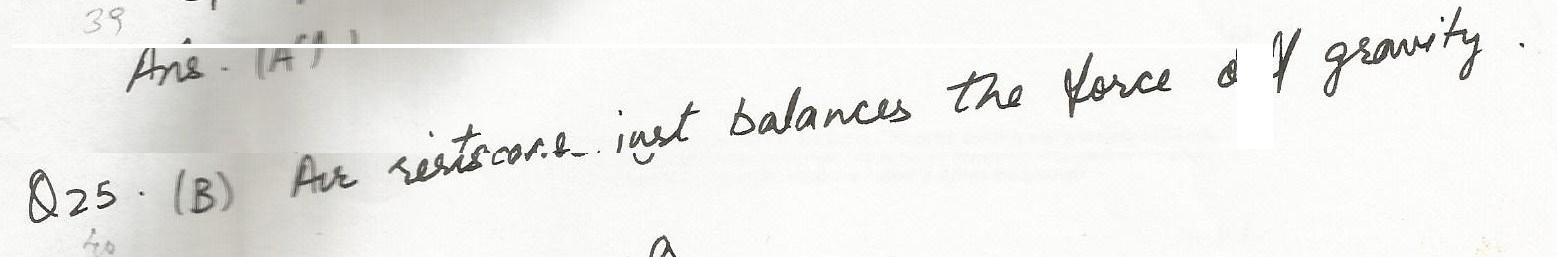6900

Physics Force & Laws Of Motion Level: Misc Level

A 1000-kg airplane moves in straight flight at constant speed. The force of air friction is 1800 N. The net force on the plane is;

a. zero

b.11800 N

c. 1800 N

d. 9800 N

e. none of these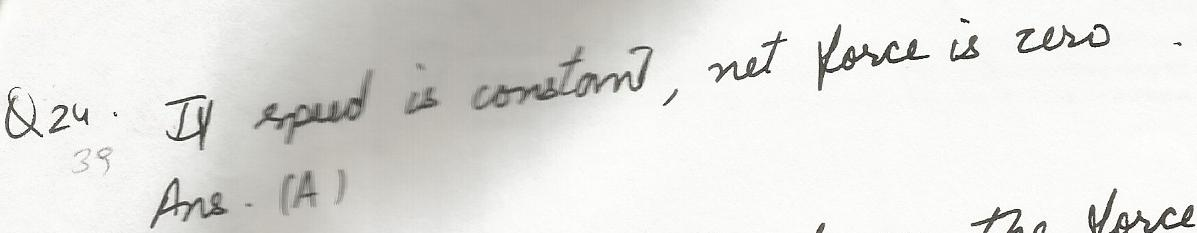6898

Physics Force & Laws Of Motion Level: Misc Level

The system shown remains at rest. Find the force of friction on the upper block;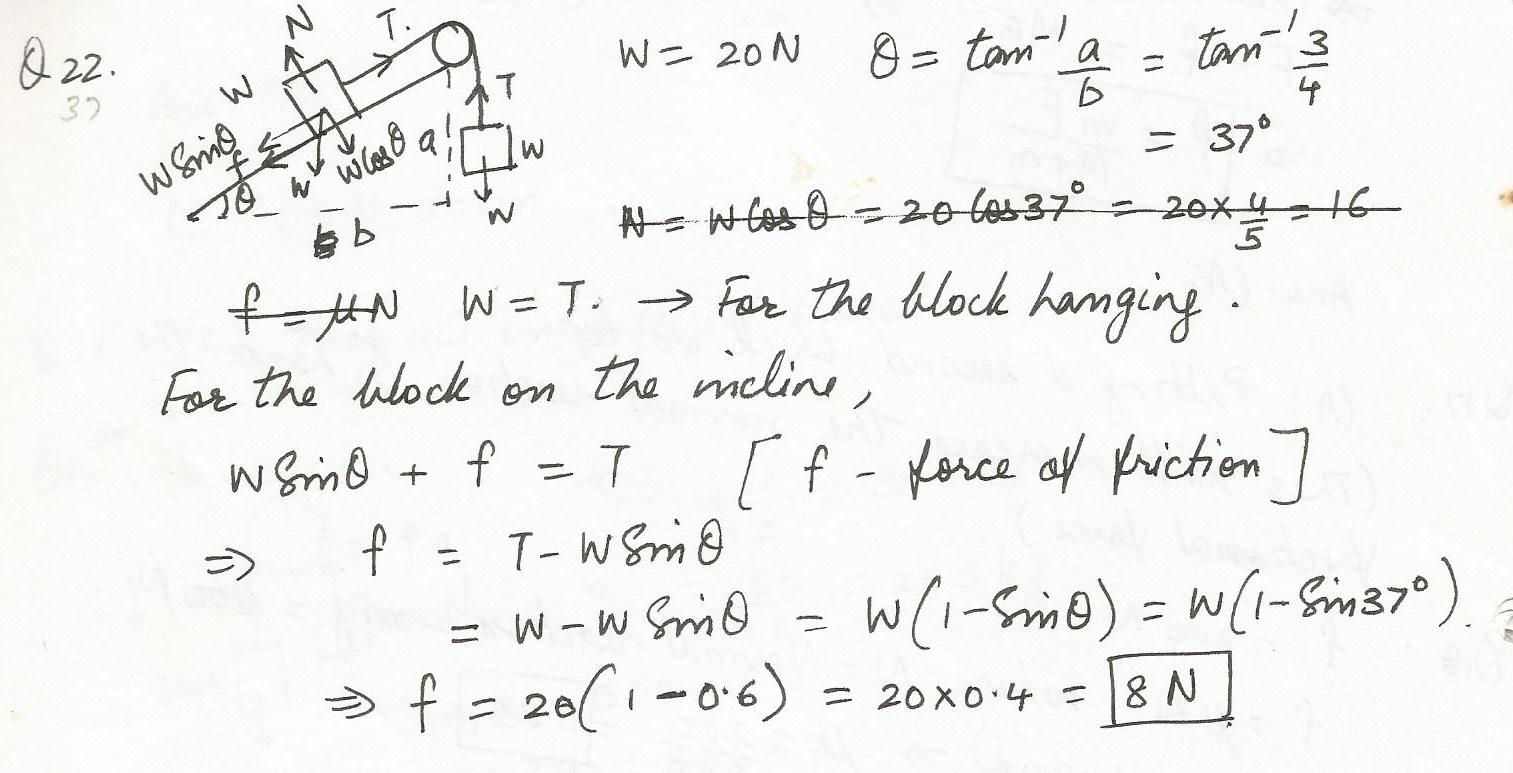6897

Physics Force & Laws Of Motion Level: Misc Level

A  block is first placed on its long side and then on its short side on the same inclined plane. The block slides down the plane on its short side but remains at rest on its long side.A possible explanation is;

a. the short side is smoother

b. the frictional force is less because the contact area is less

c.case

d. the normal force is less in the second case

e. the force of gravity is more nearly down the plane in the second case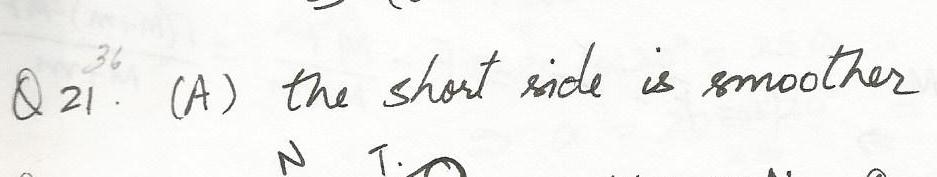6896

Physics Force & Laws Of Motion Level: Misc Level

A 400-N block  is dragged along a rough (uk=0.4) horizontal surface by an applied force c as shown. The block moves at constant velocity. Find the magnitude of F;6894

Physics Force & Laws Of Motion Level: Misc Level

A horizontal shove of at least 200-N is required to start moving a 800-N crate initially at rest on a horizontal floor. Find the coefficient of static friction is;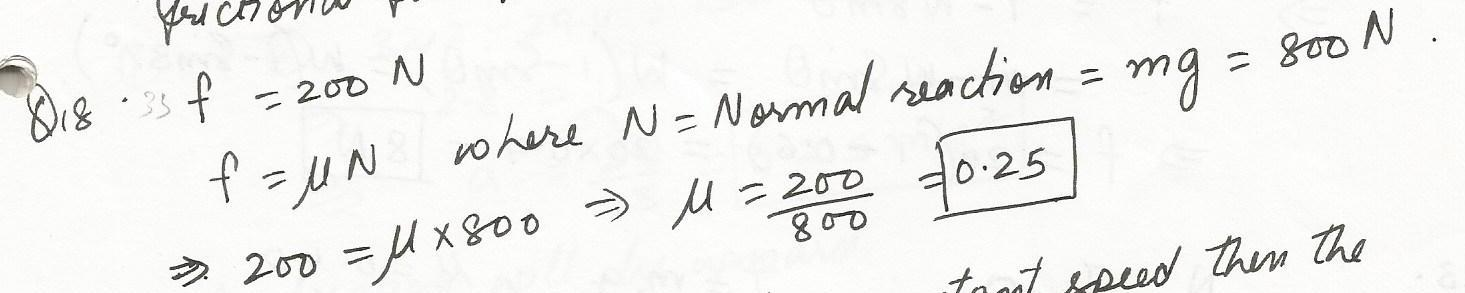6893

Physics Force & Laws Of Motion Level: Misc Level

A brick slides on a horizontal surface. Which of the following will increase the friction force on it?

a. Putting a second brick on top

b. Decreasing the surface area of contact

c. Increasing the surface area of contact

d. Decreasing the mass of the brick

e. None of the above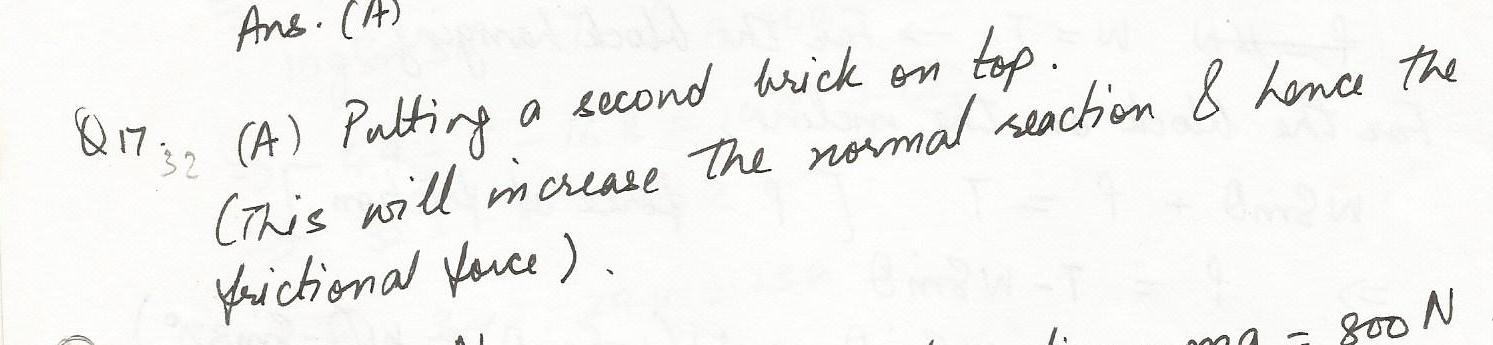6892

Physics Force & Laws Of Motion Level: Misc Level

Two blocks with masses m and M are pushed along a horizontal frictionless surface by a horizontal applied force c as shown. The magnitude of the force that either of these blocks exerts on the other is;

a. mF/ m+M)

b.mF/M

c. mF (M-m)

d. MF(M+m)

e. MF/m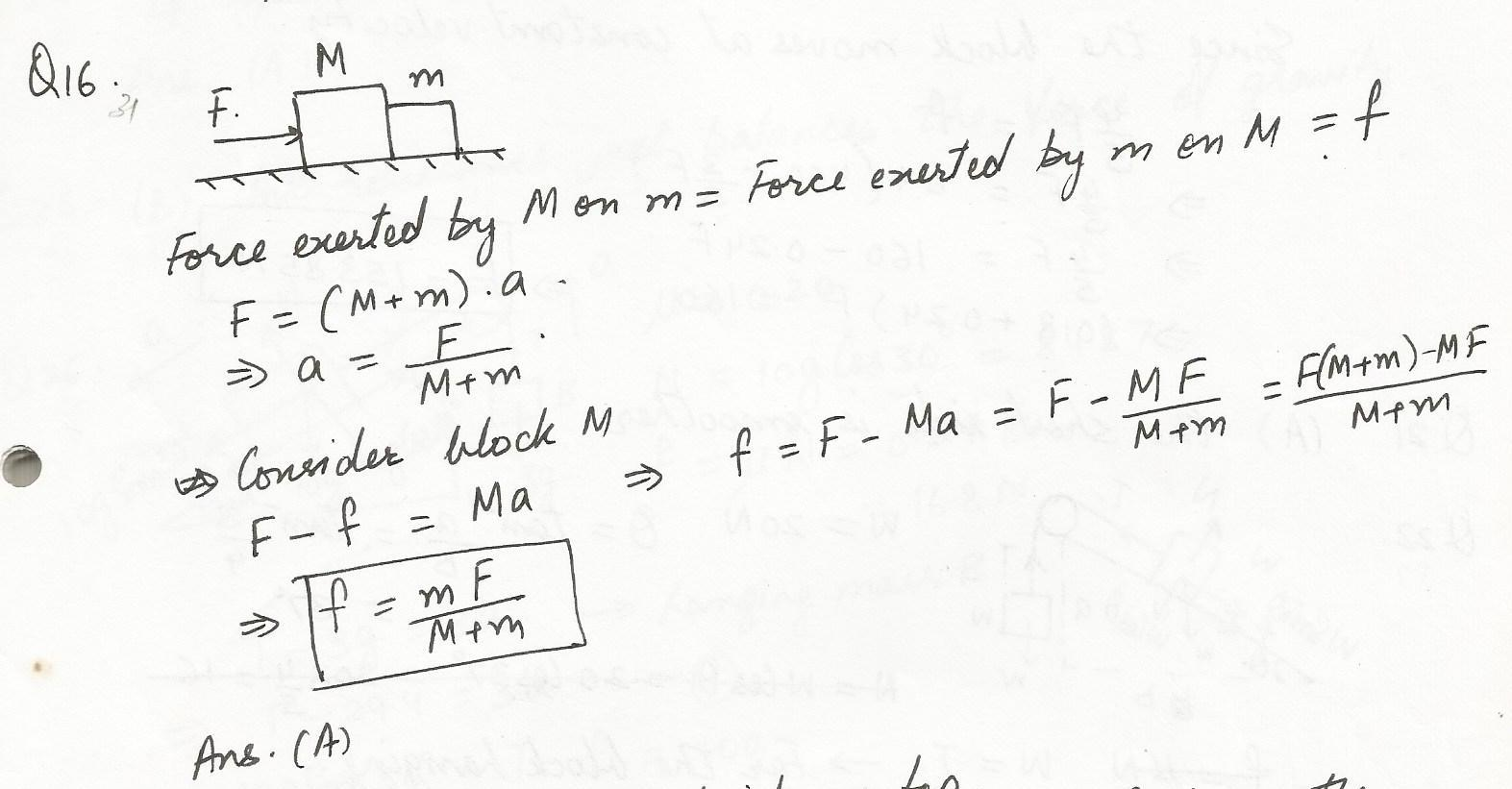6891

Physics Force & Laws Of Motion Level: Misc Level

Three blocks (A,B, C),each having mass M, are connected by strings as shown. Block C is pulled to the right by a c that causes the entire system to accelerate. Neglecting friction, find the net force acting on block B;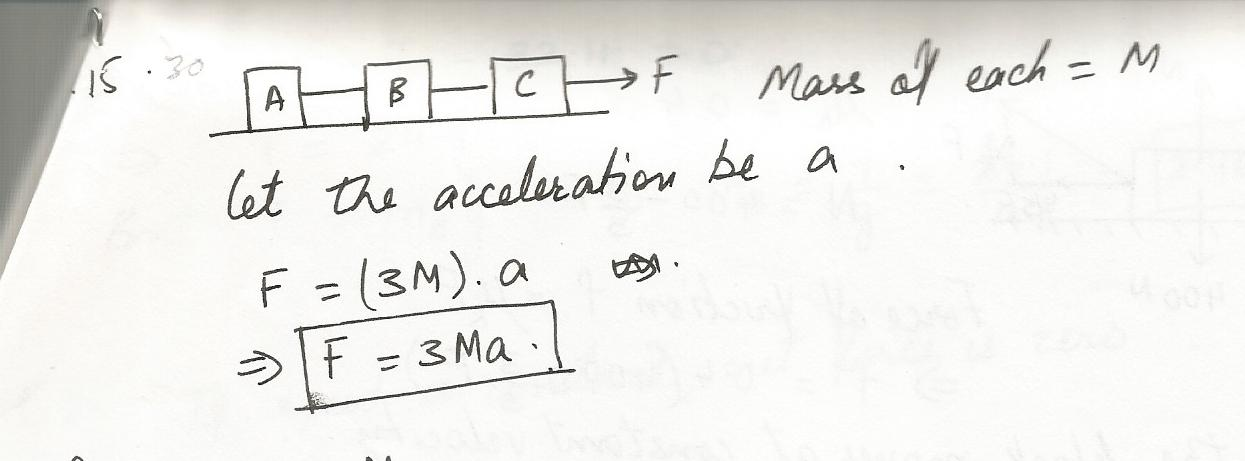6890

Physics Force & Laws Of Motion Level: Misc Level

Two blocks weighing 250 N and 350 N respectively, are connected by a string that passes over a massless pulley as shown.

Calculate the tension in the string;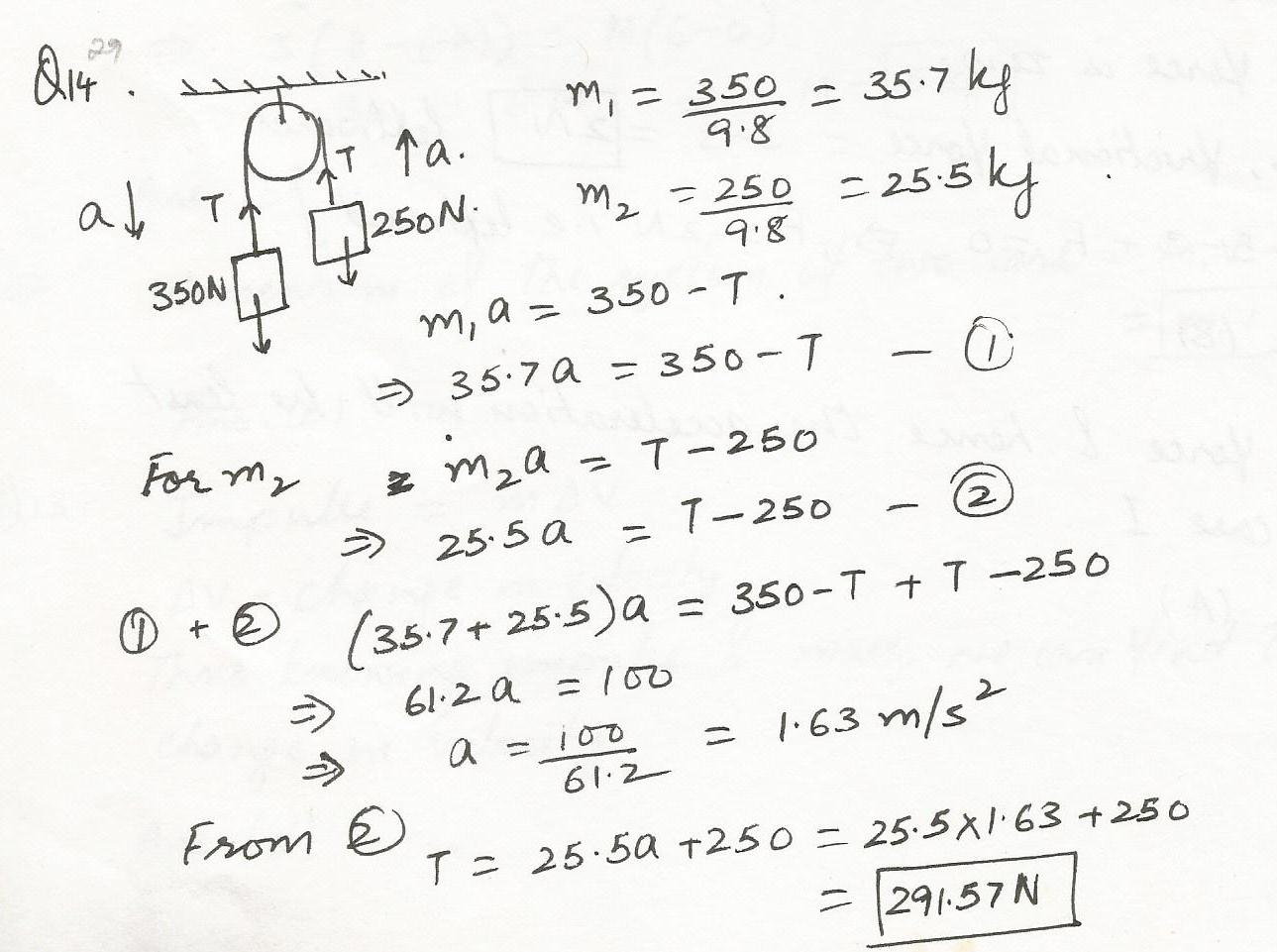6889

Physics Force & Laws Of Motion Level: Misc Level

The'' reaction'' force does not cancel the action'' force because;

a. the action force is greater than the reaction force

b. they act on different bodies

c. they are in the same direction

d. thereaction force exists only after the action force is removed

e. the reaction force is greater than the action force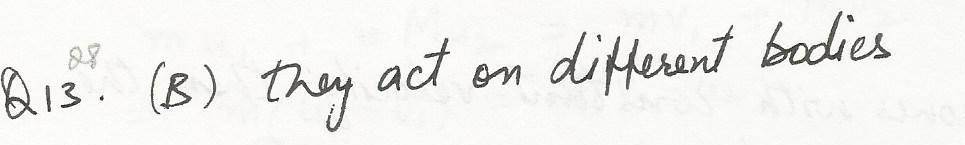6886

Physics Force & Laws Of Motion Level: Misc Level

A heavy wooden block is dragged by a force e along a rough steel plate, as shown below for cases. The magnitude of the applied force c is the same for both cases. The normal force in (ii) as compared with the normal force in  (i) is;

a. the same

b.greater

c. less

d. leass for some angles of the incline and greater for

d. others

e. less or greater, depending on the magnitude of the applied force c.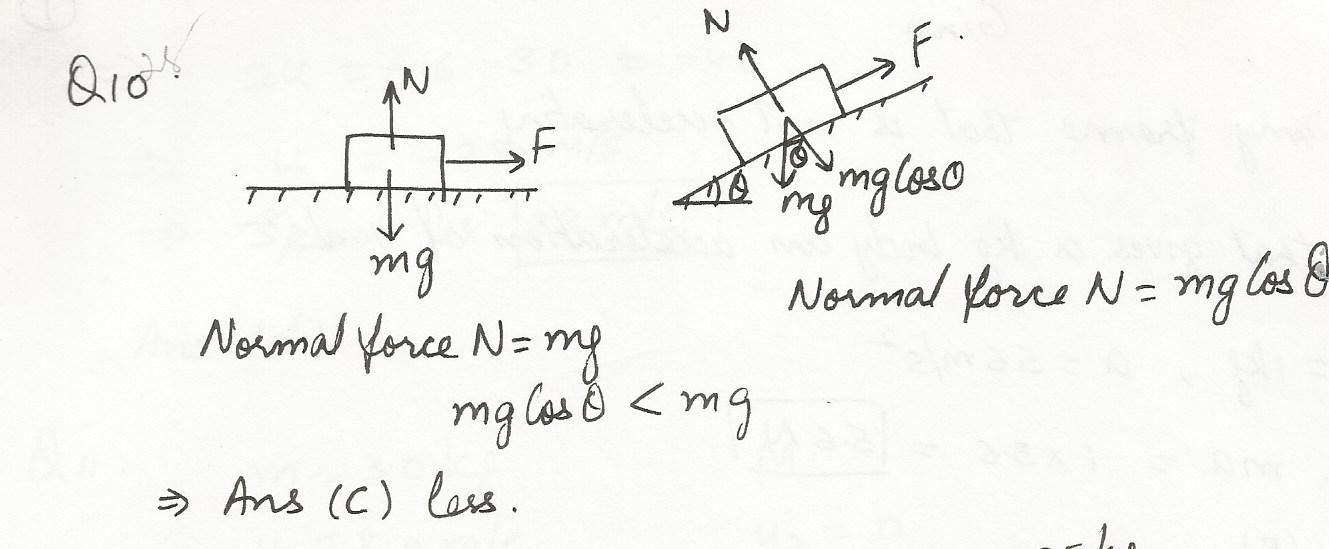6839

Physics Force & Laws Of Motion Level: Misc Level

Mass differs from weight in that;

a. all objects have weight but some lack mass

b. weight is a force and mass is not

c. the mass of an object is always more than its weight

d. mass can only be expressed in the metric system

e. there is no difference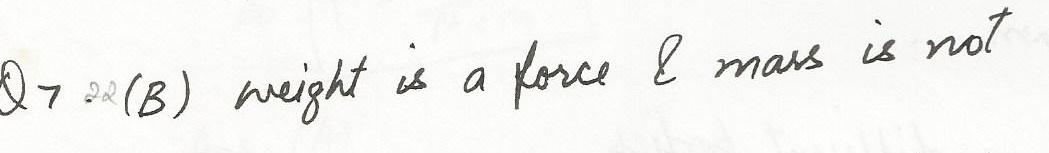6838

Physics Force & Laws Of Motion Level: Misc Level

The inertia of a body tends to cause the body to;

a. speed up

b. slow down

c. resist any change in its motion

d. fall toward the Earth

e. decelerate due to friction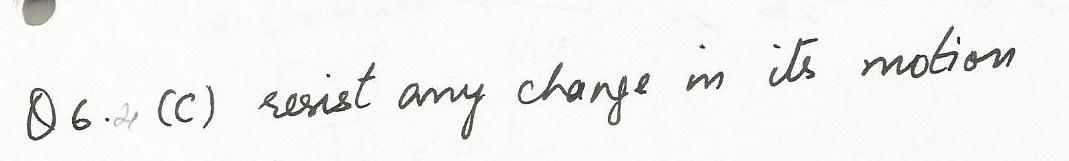6837

Physics Force & Laws Of Motion Level: Misc Level

The term" mass" refers to the same physical concept as;

a. weight

b. inertia

c. force

d, acceleration

e. volume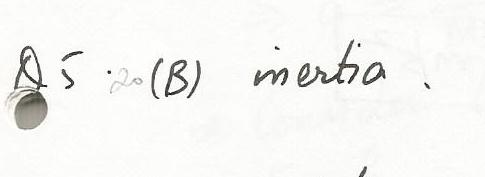Displaying 46-60 of 709 results.